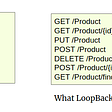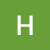# Solving Popular Algorithms: Average Salary

`s = [4000,3000,1000,2000]`
`let max = 0let min = Number.MAX_SAFE_INTEGER`
`for (let i = 0; i < s.length; i++) {    if (s[i] > max) {        max = s[i]    }    if (s[i] < min) {        min = s[i]    }}`
`//removes the max valuefor (let j = 0; j < s.length; j++) {    if (s[j] === max) {      s.splice(j, 1)    }}//removes the min valuefor (let y = 0; y < s.length; y++) {    if (s[y] === min) {      s.splice(y, 1)    }}`
`let total = 0for (let x = 0; x < s.length; x++) {    total += s[x]}return total/s.length`
`avSal = (s) => {    let max = 0    let min = Number.MAX_SAFE_INTEGER    let total = 0    //finds the max and min values    for (let i = 0; i < s.length; i++) {        if (s[i] > max) {            max = s[i]        }        if (s[i] < min) {            min = s[i]        }    }    //removes the max value    for (let j = 0; j < s.length; j++) {        if (s[j] === max) {          s.splice(j, 1)        }    }    //removes the min value    for (let y = 0; y < s.length; y++) {        if (s[y] === min) {          s.splice(y, 1)        }    }    //calculates the average    for (let x = 0; x < s.length; x++) {        total += s[x]    }    return total/s.length}`

--

--

--

## More from HopeGiometti

Love podcasts or audiobooks? Learn on the go with our new app.

## Deno vs Node — comparison## Laravel: WebAuthn the super easy way## How To Use jQuery## What is loopback and how to make loopback rest API application## Common Dilemma of using expo vs Vanilla React Native being solved.## Virtual DOM in React## An Unlikely Solution## Phase 5 — Week 4